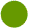P A R L A . I T A L I A N O . - . S P E A K . I T A L I A N .I M P A R A . L ' I T A L I A N O . - . L E A R N . I T A L I A N I T A L I A . - . I T A L Y M A L T A
 . - . WWW.SPEAK-ITALIAN.IT . - . . - . S P E A K . I T A L I A N . - . P A R L A . I T A L I A N O . - . L E A R N . I T A L I A N . - . I M P A R A . L ' I T A L I A N O . - . S P E A K . I T A L I A N . S T U D I O . - . . - . M A L T A . - . M I L A N O . - . G E N O V A . - . M I L A N . - . G E N O A . - . I T A L I A . - . I T A L Y . - . M A L T A . - . WWW.SPEAK-ITALIAN.IT